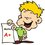Weightlessness of freely-falling body

We know, Weight (w) = Mass(m) * Acceleration due to gravity(g)

If the weight of a body is 0, either the mass or the gravitational acceleration is 0, or both are 0. But, mass of a body can never be 0. Thus the gravitational acceleration has to be 0.

But a body during free fall possesses acceleration as well as mass.

So, why is a freely-falling body weightless ?Note by Monimoy Ghosh
6 years, 5 months ago

This discussion board is a place to discuss our Daily Challenges and the math and science related to those challenges. Explanations are more than just a solution — they should explain the steps and thinking strategies that you used to obtain the solution. Comments should further the discussion of math and science.

When posting on Brilliant:

• Use the emojis to react to an explanation, whether you're congratulating a job well done , or just really confused .
• Ask specific questions about the challenge or the steps in somebody's explanation. Well-posed questions can add a lot to the discussion, but posting "I don't understand!" doesn't help anyone.
• Try to contribute something new to the discussion, whether it is an extension, generalization or other idea related to the challenge.
• Stay on topic — we're all here to learn more about math and science, not to hear about your favorite get-rich-quick scheme or current world events.

MarkdownAppears as
*italics* or _italics_ italics
**bold** or __bold__ bold

- bulleted
- list

• bulleted
• list

1. numbered
2. list

1. numbered
2. list
Note: you must add a full line of space before and after lists for them to show up correctly
paragraph 1

paragraph 2

paragraph 1

paragraph 2

> This is a quote
This is a quote
# I indented these lines
# 4 spaces, and now they show
# up as a code block.

print "hello world"
# I indented these lines
# 4 spaces, and now they show
# up as a code block.

print "hello world"
MathAppears as
Remember to wrap math in $$...$$ or $...$ to ensure proper formatting.
2 \times 3 $2 \times 3$
2^{34} $2^{34}$
a_{i-1} $a_{i-1}$
\frac{2}{3} $\frac{2}{3}$
\sqrt{2} $\sqrt{2}$
\sum_{i=1}^3 $\sum_{i=1}^3$
\sin \theta $\sin \theta$
\boxed{123} $\boxed{123}$

Sort by:

A freely-falling body isn't really "weightless." A one-pound weight free-falling in a vacuum in a lab on Earth still weighs one pound. But from the point of view of an ant on the weight (holding its little breath), there are no net forces acting on it so it experiences weightlessness.

Same for an astronaut in orbit. The orbit is shaped by the gravity of the Earth, the Moon, and the Sun. But the astronaut's net acceleration is exactly those dictated by those forces, so s/he feels "weightless."

- 6 years, 5 months ago

Thank you.

- 6 years, 4 months ago

Weightlessness occurs when you do not feel the floor. To make this clear, we will use "apparent weight".

Suppose you are in an accelerating elevator and there is conveniently a weighing scale.

Naturally, when the elevator starts accelerating, you get onto the scale and read you weight. But since the elevator is accelerating, you are actually reading your apparent weight.

Note that by Newton's third law, your apparent weight is the normal force on you. So when the elevator goes into free fall, you "lose contact" with the scale. When you read the scale, the apparent weight you read is 0, which obviously means that you are weightless.

- 6 years, 5 months ago

Thank you.

- 6 years, 4 months ago

What we mean by weight is the normal force exerted by the surface on which the block is kept on the block. So, if we are in an inertial frame the normal force would be equal to the weight of the body.( Free body diagram analysis). But, in an non-inertial frame, use free body diagram and find the normal force. This is the weight of the body. Mass is frame-independent but weight is frame-dependent.

- 6 years, 4 months ago

Thank you.

- 6 years, 4 months ago

Weight (or rather the apparent weight, i.e. the weight felt by the body) of a body is actually the reaction force that it feels from the floor on which the body is standing or the rope from which it is hanging (in the second case the reaction is tension). During a free fall a body makes no contact with anything so no question of such reaction force arises. It is actually not weightless, but feels itself to be weightless.

- 4 years, 3 months ago

Consider a body of mass 'm' with a massless rope attached to it, the rope being linked to a weighing scale. If you do the free body diagram of the given situation at hand, the scale shall read nothing but the "tension" in the rope, 'T'.

At rest, T will be exactly equal to mg.

(Visualize the weighing scale and the rope attached to the mass at all times)

When the body starts to fall freely i.e. with acceleration equal to 'g' we have a different situation here. Now the forces acting on the body shall be ----- 1) Tension T acting upwards 2) Weight mg acting downwards 3) Inertia force mg due to acceleration acting upwards as per D'Alemberts principle.

So now if you equate the above forces, we have something like this------

T +mg = mg which brings us to T=0

This means nothing but the fact that, the weighing scale won't register any reading rendering zero apparent weight although the body has mass.

- 7 months, 2 weeks ago